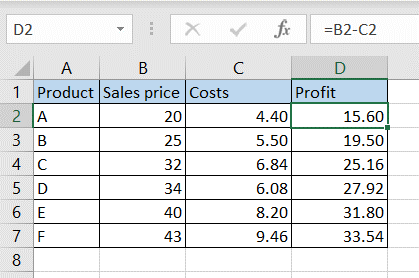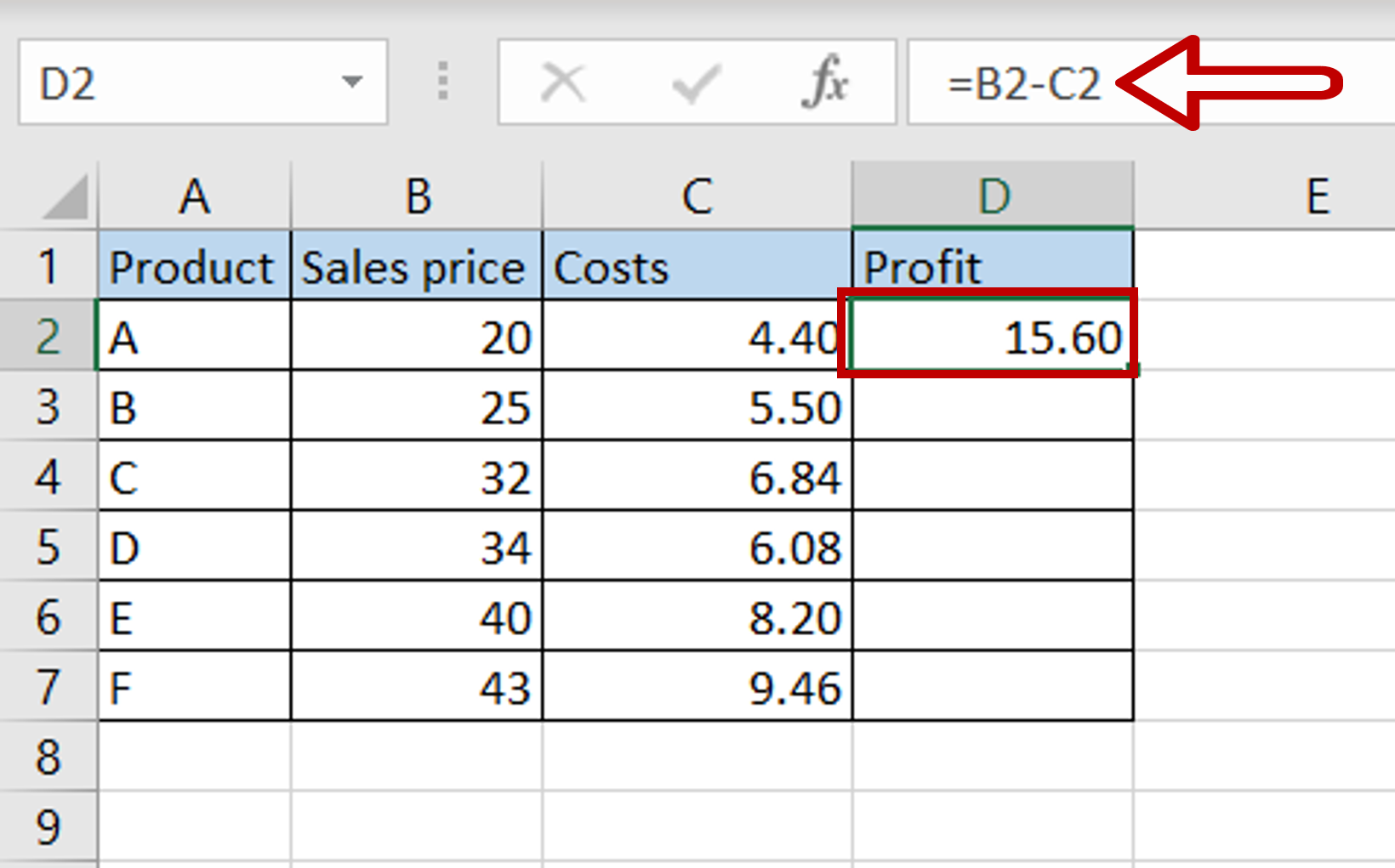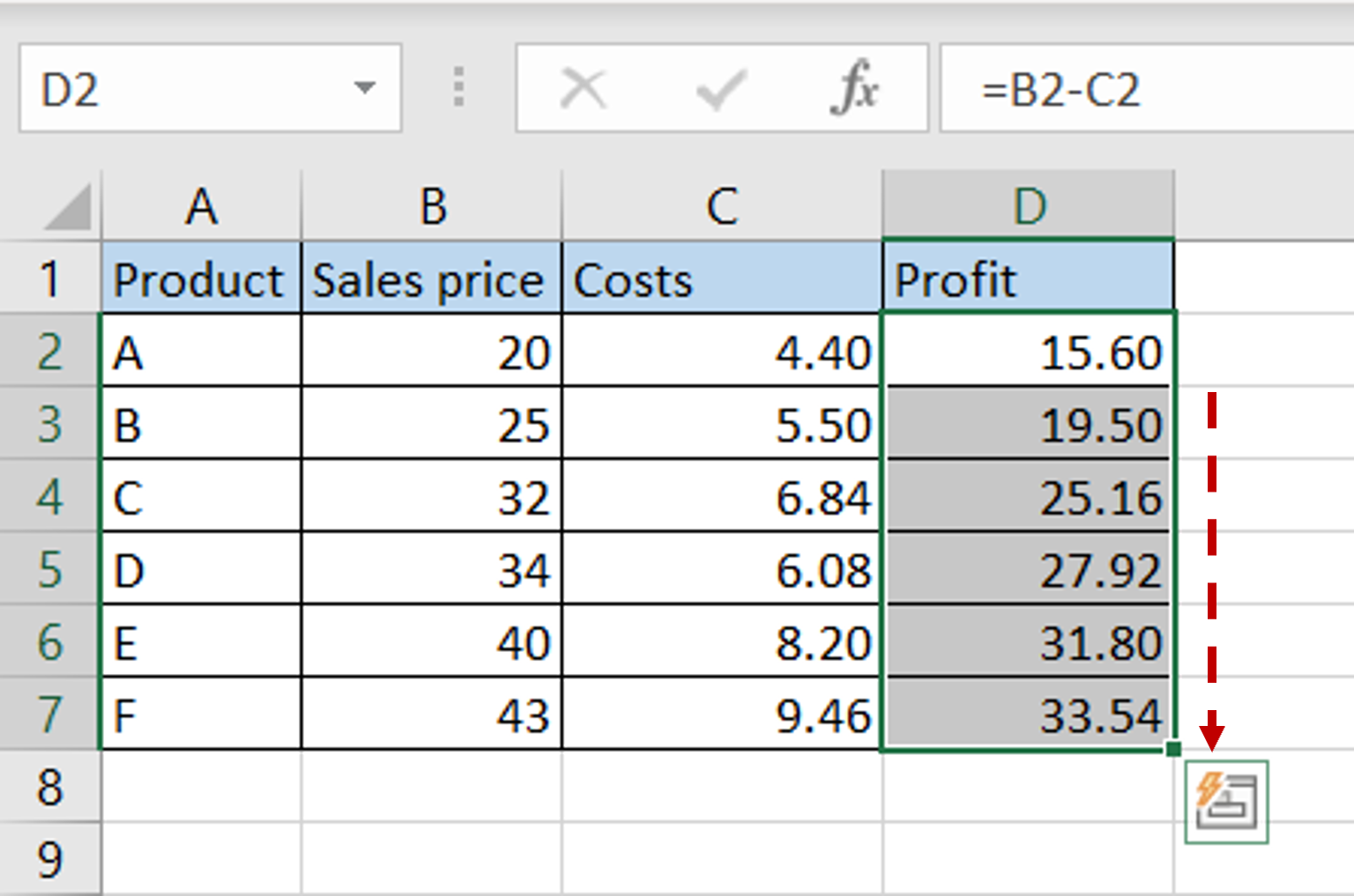# How to subtract one column from another in Excel

You can watch a video tutorial here.Excel is a great tool for performing calculations across many columns and creating complex formulas.  When creating a calculated column, you may need to subtract one column from another.

### Step 1 – Create the formula– Select the first cell in the ‘Profit’ column
– Type in the formula using cell references:
= Sales price – Costs
– Press Enter

### Step 2 – Copy the formula– Using the fill handle from the first cell, drag the formula to the remaining cells
OR
a) Select the cell with the formula and press Ctrl+C or choose Copy from the context menu (right-click)
b) Select the rest of the cells in the column and press Ctrl+V or choose Paste from the context menu (right-click)

### Step 3 – Check the result– The ‘Costs’ column is subtracted from the ‘Sales price’ column